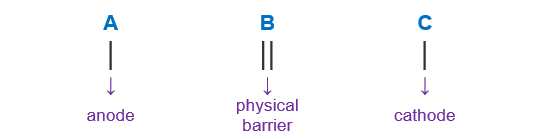# Problem: Consider the cell:Pt(s) | H2(g) | H+(aq) || Cl − (aq) | Cl 2(g) | C(s)What is serving as the cathode in this electrochemical cell?A. Cl−(aq)B. Cl2(g)C. C(s)D. Pt(s)E. H+(aq)

###### FREE Expert Solution

We’re being asked to determine the the cathode in this electrochemical cell:

Pt(s) | H2(g) | H+(aq) || Cl − (aq) | Cl 2(g) | C(s)

When writing a cell notation, we use the following format – “as easy as ABC99% (359 ratings)###### Problem Details

Consider the cell:

Pt(s) | H2(g) | H+(aq) || Cl − (aq) | Cl 2(g) | C(s)

What is serving as the cathode in this electrochemical cell?

A. Cl(aq)

B. Cl2(g)

C. C(s)

D. Pt(s)

E. H+(aq)